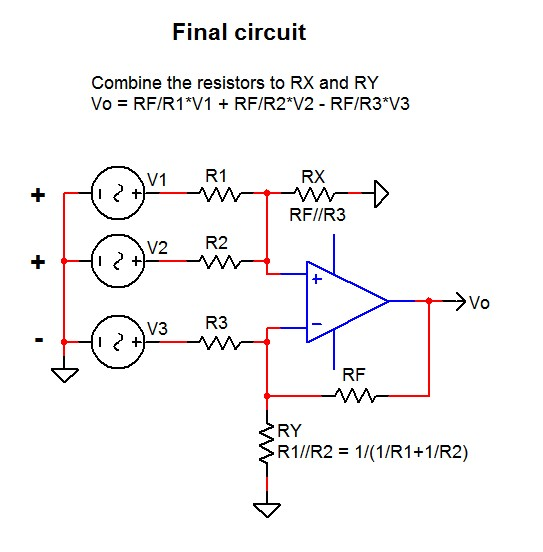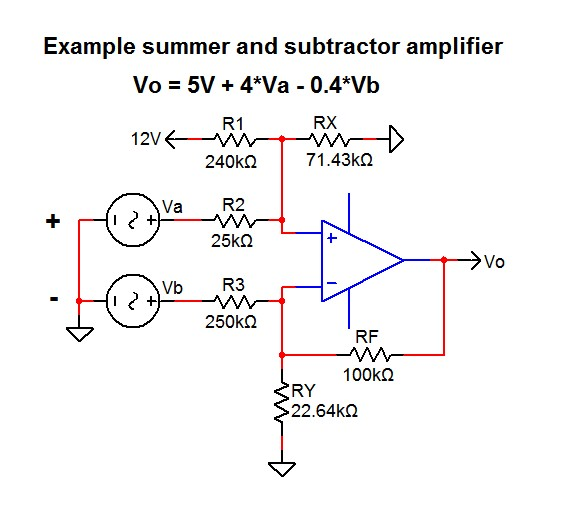#### Abstract

The design of complex op-amp circuits often turns into a puzzle and the creation of many formulas. Therefore I describe here a straightforward method which makes this task easier.
The basis of this method is the general summing amplifier, of which all these kinds of circuits can be derived:

Inverting amplifier
Non-inverting amplifier
Summing amplifier
Differential amplifier

#### General summing amplifier

The equation for 4 differential inputs is:

Vo = RF/R1*(VP1-VN1) + RF/R2*(VP2-VN2) + RF/R3*(VP3-VN3) + RF/R4*(VP4-VN4)

Note that VP and VN are always paired, we can't leave out one without the other:Simple general summing and subtracting op-amp calculation

#### Op-amp circuit example

Assume, we need an amplifier with an output offset voltage and 2 inputs, with the equation:

Vo = 5V + 4*Va - 0.4*Vb

Start with replacing the unused inputs in the general formula by 0:

Vo = RF/R1*(VP1-0) + RF/R2*(VP2-0) + RF/R3*(0-VN3)

The circuit with the unused inputs connected to ground:Summing and subtracting op-amp example

#### The final circuit

When we combine the resistors R3 and RF to RX and R1 and R2 to RY, we get the final circuit:Summing and subtracting op-amp example

The final formula is:

Vo = RF/R1*V1 + RF/R2*V2 - RF/R3*V3

Now we have to calculate the resistors in order to obtain the required formula:

Vo = 5V + 4*Va - 0.4*Vb

#### Resistor calculations

In this example, a 12V supply is available to create the 5V output offset voltage, thus V1=12V.Summing and subtracting op-amp example

Start by taking a value for RF, for example 100k.

Calculation R1
RF/R1*V1 = 5V
R1 = V1*RF/5V
R1 = 12*100k/5=240k

Calculation R2
RF/R2 = 4
R2 = RF/4
R2 = 100k/4 = 25k

Calculation R3
RF/R3 = 0.4
R3 = RF/0.4
R3 = 100k/0.4 = 250k

Calculation RX
RX = RF//R3 = 100k//250k = 71.43k

Calculation RY
RY = R1//R2 = 240k//25k = 22.64k

#### Other examples

All other similar op-amp circuits can be designed and calculated in the same way.

#### Non-inverting amplifier

Note that with this method, a non-inverting amplifier leads to a sub-optimal solution, see fig. A. Of course, fig. B is preferred here.Non-inverting amplifier

This article is new, please tell me if something has to be corrected or added.

Do you have any comments about the website? Please let me know.